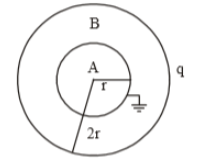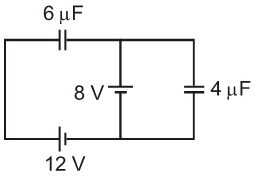The capacitance of a parallel plate capacitor is C. If a dielectric slab of thickness equal to one-fourth of the plate separation and dielectric constant K is inserted between the plates, then new capacitance become

1. $\frac{KC}{2\left(K+1\right)}$

2. $\frac{2KC}{K+1}$

3. $\frac{5KC}{4K+1}$

4. $\frac{4KC}{3K+1}$

Concept Questions :-

Dielectrics
High Yielding Test Series + Question Bank - NEET 2020

Difficulty Level:

The electric potential at a point in space due to charge Q is Q V. The value of an electric field at that point will be

1.  $\frac{\mathrm{Q}}{9}×{10}^{12}\frac{\mathrm{N}}{\mathrm{C}}$

2.  $\frac{\mathrm{Q}}{9}×{10}^{15}\frac{\mathrm{N}}{\mathrm{C}}$

3.  $\frac{\mathrm{Q}}{9}×{10}^{9}\frac{\mathrm{N}}{\mathrm{C}}$

4.

Concept Questions :-

Relation between field and potential
High Yielding Test Series + Question Bank - NEET 2020

Difficulty Level:

The electric potential at a point at distance 'r' from a short dipole is proportional to

(1) ${r}^{2}$

(2) ${\mathrm{r}}^{-1}$

(3) ${r}^{-2}$

(4) ${r}^{1}$

Concept Questions :-

Electric potential
High Yielding Test Series + Question Bank - NEET 2020

Difficulty Level:

A hollow charged metal spherical shell has radius R. If the potential difference between its surface and a point at a distance 3R from the center is V, then the value of electric field intensity at a point at distance 4R from the center is

1.  $\frac{3\mathrm{V}}{19\mathrm{R}}$

2.  $\frac{\mathrm{V}}{6\mathrm{R}}$

3.  $\frac{3\mathrm{V}}{32\mathrm{R}}$

4.  $\frac{3\mathrm{V}}{16\mathrm{R}}$

Concept Questions :-

Relation between field and potential
High Yielding Test Series + Question Bank - NEET 2020

Difficulty Level:

A metallic sphere is given some charge. Electric energy is stored

(1) Only inside the sphere

(2) Only outside the sphere

(3) Both inside and outside

(4) Neither inside nor outside

Concept Questions :-

Electric potential energy
High Yielding Test Series + Question Bank - NEET 2020

Difficulty Level:

Capacitors  are connected in series across a source of emf 20KV. The potential difference across ${C}_{1}$ will be

(1) 5 KV

(2) 15 KV

(3) 10 KV

(4) 20 KV

Concept Questions :-

Combination of capactiors
High Yielding Test Series + Question Bank - NEET 2020

Difficulty Level:

The equivalent capacitance between A and B is as the given figure:(1) $16\mathrm{\pi }{\in }_{0}\mathrm{r}$

(2) $4\mathrm{\pi }{\in }_{0}\mathrm{r}$

(3) $8\mathrm{\pi }{\in }_{0}\mathrm{r}$

(4) None of these

Concept Questions :-

Combination of capactiors
High Yielding Test Series + Question Bank - NEET 2020

Difficulty Level:

Two metallic spheres of radii 2cm and 3cm are given charges 6mC and 4mC respectively. The final charge on the smaller sphere will be if they are connected by a conducting wire

(1) 4mC

(2) 6mC

(3) 5mC

(4) 10mC

Concept Questions :-

Electric potential
High Yielding Test Series + Question Bank - NEET 2020

Difficulty Level:

When a proton at rest is accelerated by a potential difference V, its speed is found to be v. The speed of an $\alpha$ particle when accelerated by the same potential difference from rest will be

(1) v

(2) $\frac{v}{\sqrt{2}}$

(3) $v\sqrt{2}$

(4) 2v

Concept Questions :-

Electric potential energy
High Yielding Test Series + Question Bank - NEET 2020

Difficulty Level:

In the circuit shown in figure, energy stored in 6$\mathrm{\mu F}$ capacitor will be1.

2.

3.

4.

Concept Questions :-

Energy stored in capacitor